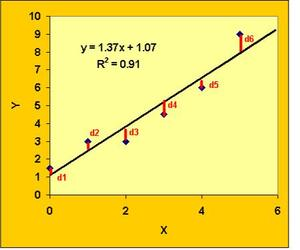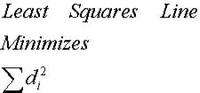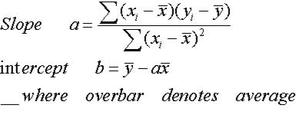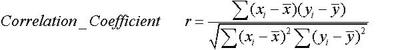|

# Linear Regression

• a brief outline of linear regression;
• two pdf file link that describe how to use MS Excel functions Trendline and LINEST;
• links to interactive online tools for regression;
• links to interactive online regression tutorials;
• This link to the activity (Statistics of US Historical Climate Network) which uses Excel to estimate the trend in the long term temperature record for any Climate Network station.
• other related references.

Finding the best linear fit between two paired variables is very useful in many geoscience applications. For example, one might want to estimate the increase in global temperature per decade by performing a linear regression of the global mean temperature on time. As another example, by plotting rock permeability versus density for a particular formation we can get a visual feeling for the possible relationship between these two variables. Performing a least squares linear regression of density on porosity provides an objective method to quantify the linear relationship between these measurements. Often using one's subjective judgment to draw a "best fit" line through the data can also serve as a useful first estimate in the field.The basic idea of any least squares fit whether it is a linear least squares fit or a polynomial fit is to find the curve which minimizes the sum of the vertical distances squared between all data point and the least squares line. The square of each distance is used to easily ignore the sign of this distance. Using Trendline command in MS Excel (Acrobat (PDF) 163kB Jul30 04) discusses how to get the least squares fit shown above within Microsoft Excel. The file LINEST Help (Acrobat (PDF) 169kB Aug3 04) describes how one can use the MS Excel LINEST function to obtain additional statistical information including the estimate of confidence intervals for the slope and intercept.

The linear least square line has a slope a and intercept b given byThe correlation coefficient r varies between -1 and +1. The square of the correlation coefficient represents the fraction of the total variance explained by regression (0 to 1.0 indicating absolutely no linear relationship to a perfect linear fit).There are many textbooks and websites that present the basic theory, limitations, and estimate of confidence of least squares linear regression. We will not repeat that discussion here but simply provide links to online regression tools that you and your students may find useful when working with data. Most spreadsheet programs and calculators also have built in function to calculate the slope and intercept of the least squares fit between data points plotted on an X-Y scatter plot.

Tutorials

• Linear regression Applet (www.math.csusb.edu/faculty/stanton/probstat/regression.html) describes estimates, residuals, and confidence bands (link unavailable).

References:

• An excellent text on statistical applications with many clear numerical examples. G.S. Snedecor and W.G.Cochran, 1989. Statistical Methods, Eighth Edition from the Iowa University Press.
• Hyperstat has a good basic discussion of the estimate confidence intervals for correlation and regression. See also t-test